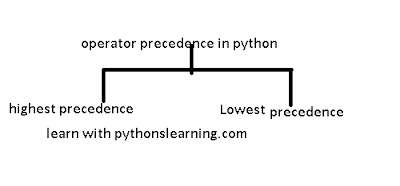# operator precedence in python :

Welcome everyone, today's we are going to see what is operator precedence in python.
So let's start:
There are Two type Of operator Precedence
• highest precedence
• highest precedenceoperator precedence in python- operator associativity

following table lists show operator from highest  to lowest precedence.

## ``` OperatorDescription [**]This is Exponentiation precedence it's raise to the power [~ + -]This is Complement, unary plus and minus precedence  [* / % //]This is Multiply, divide, modulo and floor division precedence [+ -]This is Addition and subtraction precedence [>> <<]This is Right and left bitwise shift precedence [&]This is Bitwise 'AND'td> precedence [^ |]This is Bitwise exclusive `OR' and regular `OR' precedence [<= < > >=]This is Comparison operators precedence [<> == !=]This is Equality operators precedence [= %= /= //= -= += *= **=]This is Assignment operators precedence [is is not]This is Identity operators precedence [in not in]This is Membership operators precedence [not or and]This is Logical operators precedence ```

For example, z = 4 + 5 * 6; here, z is assigned 34, not be 54 reason is because operator * has higher precedence than +operator, so it first multiplies 5*6 and then adds into 4.Operator precedence affect how an expression is evaluated in  arthi.

### Example

```#programming with pythonslearning.com

p = 10
z = 20
r = 30
s = 5
pa = 0

pa = (p + z) * r / s       #( 10 + 20 )* 30/ 5
print "ans of (p + z) * r / s is shown ",  pa

pa = ((p + z) * r) / s     # ((10 + 20 )*30)/ 5
print "ans of ((p + z) * r) / s is shown ",  pa

pa = (p + z) * (r / s);    # (10+20) * (30/5)
print "ans of (p + z) * (r / s) is shown ",  pa

pa = p + (z * r) / s;      #  10 + (20*30)/5
print "ans of p + (z * r) / s is shown ", pa```
After the execute the above programs, it produce the following outcome results −
```ans of (p + z) * r / s is shown 180
ans of ((p + z) * r) / s is shown 180
ans of (p + z) * (r / s) is shown 180
ans of p + (z * r) / s is shown 130```

## Python operator associativity

If  two or more operator have the same precedence, then associativity  of function defines the order of operation.

#### What does the associativity mean in Python?

The associativity  is the containing multiple operators of the same precedence. Almost all operator  support the left-to-right associativity except the exponent (**).
Some FAQ about operator precedence in python

### 1.  What is operator precedence in Python?

To evaluate the types of expressions the rule make precedence in Python.There can be always more than one operators in an expression.
2. Which operator has highest precedence in Python?
For example we know multiplication and division have a higher precedence than addition and subtraction operator.
```
[**]This is Exponentiation precedence it's raise to the power
[~ + -]This is Complement, unary plus and minus precedence
[* / % //]This is Multiply, divide, modulo and floor division precedence
[+ -]This is Addition and subtraction precedence
[>> <<]This is Right and left bitwise shift precedence
[&]This is Bitwise 'AND'td> precedence
[^ |]This is Bitwise exclusive `OR' and regular `OR' precedence

```

#### 3. Which operator has the lowest precedence in Python?

```
[<= < > >=]This is Comparison operators precedence
[<> == !=]This is Equality operators precedence
[= %= /= //= -= += *= **=]This is Assignment operators precedence
[is is not]This is Identity operators precedence
[in not in]This is Membership operators precedence
[not or and]This is Logical operators precedence

```
Tags: operator precedence in python- operator associativity ,lowest precedence,highest precedence.
For the similar post like python operator click on operators in python.
if you want to learn python for free then click on python tutorials point.
BEST OF LUCK!!!
Previous
Next Post »

### comparison python vs r vs sas vs spss

python  vs r vs sas vs spss : Welcome everyone, Today we will see comparison between  r vs python vs sas vs spss . So Let's start : ...